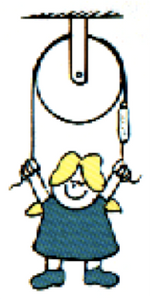# Net Force, Statics, and Equilibrium

Of course, when we measure the weight of an object on a scale, it is NOT in free fall.

That is because gravity is not the only force on the object.

In the example of a spring scale, gravity is pulling the object down, but tension in the spring or string is pulling the object up!  Since the object is stationary or STATIC, the NET FORCE must be equal to zero.   An object that is static is said to be in EQUILIBRIUM.

If the NET FORCE were not zero, the object would be accelerating up or down, and would NOT be STATIC.

As we continue this discussion of STATICS and forces, we do not have to limit ourselves to gravity.  For instance, suppose there are two hamsters pushing on a flask.  If the Ninja Hamster is pushing with 200 N to the left, and the Science Hamster is pushing with 200N to the Right, then the Net Force is zero, and the flask is static.  However, using his special powers, the Ninja Hamster now pushes with 300 N.   The NET Force is no longer zero.  The NET Force is now 100N to the right.  The Flask will now accelerate. It is no longer in Equilibrium.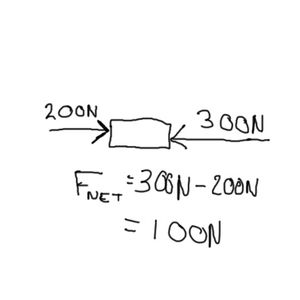Let us return to the gravity examples. Now if I were holding my object up with two strings, the scale would not measure the full weight of the object.  Each spring scale would only measure half of the weight. In this case, the NET FORCE still equals zero.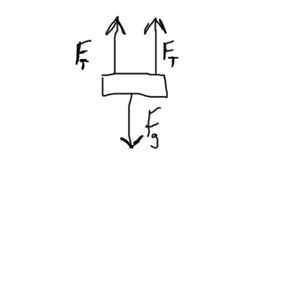Let us do an example with STATICS.  If we had some window washers on the Prudential Center.  Mike has a weight of 700 Newtons.  Ryan has a weight of 600 Newtons.  The platform they are sitting on has a weight of 2000 Newtons.  What is the tension in each string holding them up?

First we calculate the total force downwards.  2000N + 800N + 600N = 3400 N.  Since they are static, there must be 3400N upwards.  As we have two ropes, each rope must support half the weight.  So the tension in each rope is 1700N.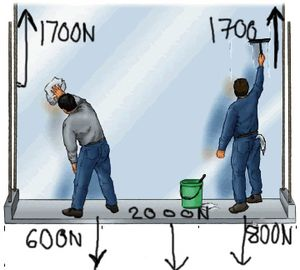In the case of a book resting on a table, gravity is pulling the book down.  However, a natural restoring force, known as the NORMAL force, pushes the book back up.  The word NORMAL means perpendicular to a surface.  For instance, in geometry, in a circle, a radius is always normal to the edge of the circle.  The NORMAL force is only as strong as the gravity pushing down on it.  If the situation is static, the NET FORCE is zero. If the table could not supply enough NORMAL force the table would collapse and gravity would win out. If the NORMAL force were greater, then the object would fly back up into the air.  A simple sketch like this is called a FREE BODY DIAGRAM.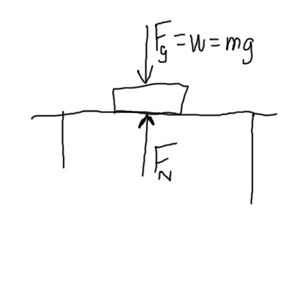It is important to reemphasize that the NORMAL force is always perpendicular to the surface.  It is NOT always pointed up.  For instance, if I were to try pushing the book through a wall, it does not easily pass through the wall.  I am pushing the book to the right.  The wall is exerting  a normal force to the left.  The Free Body Diagram looks like this.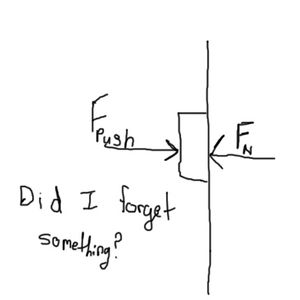Even on an inclined plane, the Normal force is still perpendicular to the surface.  Why doesn’t the hamster slide down the ramp?  Friction prevents the hamster from sliding down the ramp.  Friction is a force which is always paralallel the the surface. If we draw the forces on this hamster, gravity is always down.  Friction is parallel to the ramp, and the normal force is perpendicular.  Even through these forece are not collinear, they will still cancel out.  This is called vector addition. We need some trigonometry to solve this problem. The Free Body Diagram looks like this.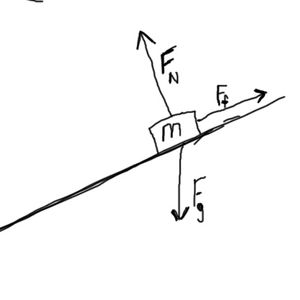Another example of this is if we have strings.  Returning to our sign painters.

When we have two vertical strings, then tension in each string is exactly half the weight.  However, as the strings are stretched to angles, that tension increases.  That is because the strings are not only supporting the weight of the object.  They are also pulling against each other. So the spring scales actually measure a greater tension.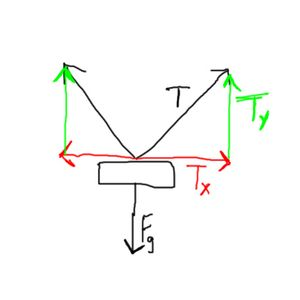Pulleys can be used in several ways.  They can change the direction of the tension force.  They can also distribute the tension in one string among several strings.  It should be noted that in physics, there is no such thing as a free lunch.  Although you might not have to pull with as much force to lift a heavy weight, you need to pull twice as much rope to attain a certain height.